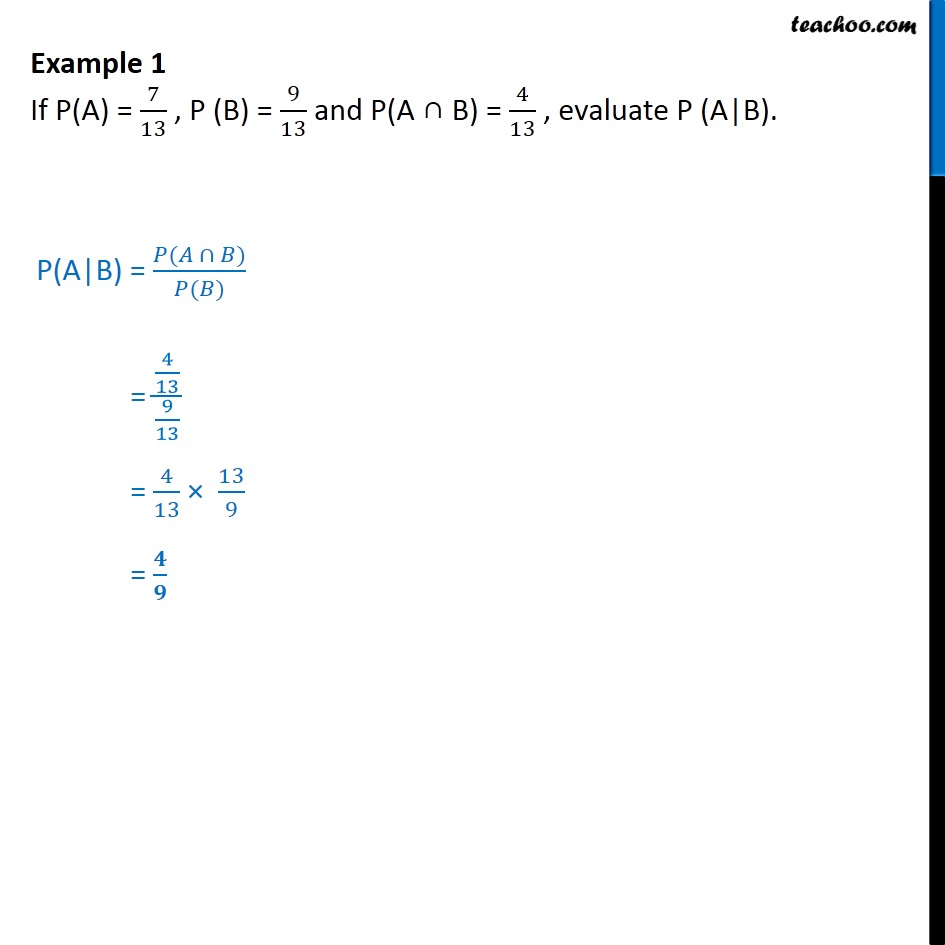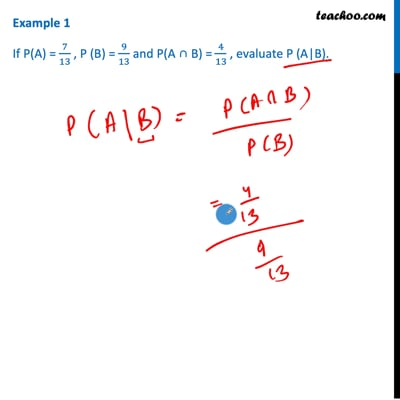Conditional Probability - Values given

Chapter 13 Class 12 Probability
Concept wiseThis video is only available for Teachoo black users

Solve all your doubts with Teachoo Black (new monthly pack available now!)

### Transcript

Example 1 - Chapter 13 Class 12 Probability - NCERT If P(A) = 7/13 , P(B) = 9/13 and P(A ∩ B) = 4/13 , evaluate P (A|B). P(A|B) = P(A ∩ B)/ P(B) = 4/13 / 9/13 = 4/13 x 13/9 = 4/9